Automation Guide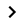Action-based testing languageThe test languageFunctionsThe requested page is not available for selected version.

# Date functions

Date functions compute and convert Gregorian dates to and from Julian day numbers.

“Julian day” refers to a continuous count of days since November 24, 4714 BC. For example, the Julian day number 2,451,545 is equivalent to a Gregorian date of January 1, 2000. The Gregorian calendar is also known as the Western calendar. The benefit of using the Julian day number is that simple arithmetic operations such as addition, subtraction, multiplication, and division can easily be performed on Julian dates. For instance, the following line converts January 15, 2012 to a Julian day number, adds 5 days, and converts the result back to a Gregorian date (January 20, 2012).

``````                     name       value
local variable       d          #fd (d (2012, 01, 15) + 5)
``````

The action line above assigns the value 2012-01-20 to local variable d.

d(yyyy,mm,dd)
Convert a Gregorian date in numeric form to its equivalent Julian day number.

today()
Convert today’s date to its equivalent Julian day number.

fj(date)
Convert a specific Gregorian date to its equivalent Julian day number.

year(Julian number)
Return the Gregorian year from a Julian date number.

month(Julian number)
Return the Gregorian month number from a Julian date number.

day(Julian number)
Return the Gregorian day of month number from a Julian date number.

plusy(Julian number, number of year)
Add a specified number of years to a given Julian date number, then return the result as a Julian number.

plusm(Julian number, number of month)
Add a specified number of months to a given Julian date number, then return the result as a Julian number.

plusd(Julian number, number of day)
Add a specified number of days to a given Julian date number, then return the result as a Julian number.

plusbd(Julian number, number of business day)
Add a specified number of business days to a given Julian date number, then return the result as a Julian number.

fd(Julian number)
Convert a Julian date number to its equivalent Gregorian date.

weekday(Julian number)
Return the day of week numeric value for a given Julian number.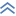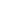1730 S. Amphlett Blvd. Suite 200, San Mateo, CA 94402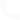Tel: +1(800) 322-0333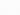Email: [email protected]